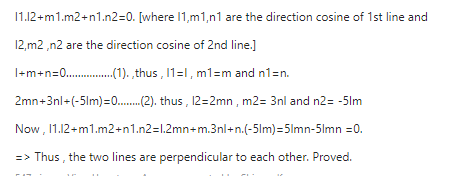# Show that the lines whose direction cosines are given by l+m+n=0 and 2mn+3ln-5lm=0 are perpendicular to each otherSaurabh Koranglekar
3 years ago3 years ago

Condition : if two lines are perpendicular to each other is :-

l1.l2+m1.m2+n1.n2=0. [where l1,m1,n1 are the direction cosine of 1st line and

l2,m2 ,n2 are the direction cosine of 2nd line.]

l+m+n=0…………….(1). ,thus , l1=l , m1=m and n1=n.

2mn+3nl+(-5lm)=0……..(2). thus , l2=2mn , m2= 3nl and n2= -5lm

Now , l1.l2+m1.m2+n1.n2=l.2mn+m.3nl+n.(-5lm)=5lmn-5lmn =0.

=> Thus , the two lines are perpendicular to each other. Proved.